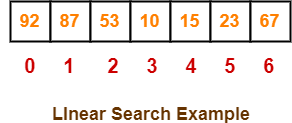# Linear Search Algorithm | Example | Time Complexity

## Searching-

• Searching is a process of finding a particular element among several given elements.
• The search is successful if the required element is found.
• Otherwise, the search is unsuccessful.

## Searching Algorithms-

Searching Algorithms are a family of algorithms used for the purpose of searching.

The searching of an element in the given array may be carried out in the following two ways-1. Linear Search
2. Binary Search

## Linear Search-

• Linear Search is the simplest searching algorithm.
• It traverses the array sequentially to locate the required element.
• It searches for an element by comparing it with each element of the array one by one.
• So, it is also called as Sequential Search.

Linear Search Algorithm is applied when-

• No information is given about the array.
• The given array is unsorted or the elements are unordered.
• The list of data items is smaller.

## Linear Search Algorithm-

Consider-

• There is a linear array ‘a’ of size ‘n’.
• Linear search algorithm is being used to search an element ‘item’ in this linear array.
• If search ends in success, it sets loc to the index of the element otherwise it sets loc to -1.

Then, Linear Search Algorithm is as follows-

### Linear_Search (a , n , item , loc)

```Begin
for i = 0 to (n - 1) by 1 do
if (a[i] = item) then
set loc = i
Exit
endif
endfor
set loc = -1
End```

## Time Complexity Analysis-

Linear Search time complexity analysis is done below-

### Best case-

In the best possible case,

• The element being searched may be found at the first position.
• In this case, the search terminates in success with just one comparison.
• Thus in best case, linear search algorithm takes O(1) operations.

### Worst Case-

In the worst possible case,

• The element being searched may be present at the last position or not present in the array at all.
• In the former case, the search terminates in success with n comparisons.
• In the later case, the search terminates in failure with n comparisons.
• Thus in worst case, linear search algorithm takes O(n) operations.

Thus, we have-

 Time Complexity of Linear Search Algorithm is O(n). Here, n is the number of elements in the linear array.

## Linear Search Efficiency-

• Linear Search is less efficient when compared with other algorithms like Binary Search & Hash tables.
• The other algorithms allow significantly faster searching.

## Linear Search Example-

Consider-

• We are given the following linear array.
• Element 15 has to be searched in it using Linear Search Algorithm.Now,

• Linear Search algorithm compares element 15 with all the elements of the array one by one.
• It continues searching until either the element 15 is found or all the elements are searched.

Linear Search Algorithm works in the following steps-

### Step-01:

• It compares element 15 with the 1st element 92.
• So, it moves to the next element.

### Step-02:

• It compares element 15 with the 2nd element 87.
• So, it moves to the next element.

### Step-03:

• It compares element 15 with the 3rd element 53.
• So, it moves to the next element.

### Step-04:

• It compares element 15 with the 4th element 10.
• So, it moves to the next element.

### Step-05:

• It compares element 15 with the 5th element 15.
• Since 15 = 15, so required element is found.
• Now, it stops the comparison and returns index 4 at which element 15 is present.

To gain better understanding about Linear Search Algorithm,

Watch this Video Lecture

Next Article- Binary Search

Get more notes and other study material of Design and Analysis of Algorithms.

Watch video lectures by visiting our YouTube channel LearnVidFun.

SummaryArticle Name
Linear Search Algorithm | Example | Time Complexity
Description
Linear Search Algorithm is the simplest searching algorithm. Linear Search Algorithm Example & Time Complexity. Linear Search Algorithm searches for an element by comparing it with each element of the array.
Author
Publisher Name
Gate Vidyalay
Publisher Logo